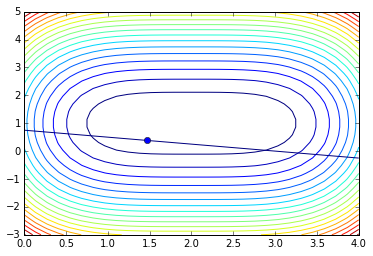(which is just using Newton on a Lagrangian)

In :
import numpy as np
import numpy.linalg as la


Here's an objective function $f$ and a constraint $g(x)=0$:

In :
def f(vec):
x = vec
y = vec
return (x-2)**4 + 2*(y-1)**2

def g(vec):
x = vec
y = vec
return x + 4*y - 3


Now define the Lagrangian, its gradient, and its Hessian:

In :
def L(vec):
lam = vec
return f(vec) + lam * g(vec)

x = vec
y = vec
lam = vec

return np.array([
4*(x-2)**3 + lam,
4*(y-1) + 4*lam,
x+4*y-3
])

def hess_L(vec):
x = vec
y = vec
lam = vec

return np.array([
[12*(x-2)**2, 0, 1],
[0, 4, 4],
[1, 4, 0]
])


At this point, we only need to find an unconstrained minimum of the Lagrangian!

Let's fix a starting vector vec:

In :
vec = np.zeros(3)


Implement Newton and run this cell in place a number of times (Ctrl-Enter):

In :
vec = vec - la.solve(hess_L(vec), grad_L(vec))
vec

Out:
array([ 1.46398989,  0.38400253,  0.61599747])

Let's first check that we satisfy the constraint:

In :
g(vec)

Out:
0.0

Next, let's look at a plot:

In :
import matplotlib.pyplot as pt
x, y = np.mgrid[0:4:30j, -3:5:30j]
pt.contour(x, y, f(np.array([x,y])), 20)
pt.contour(x, y, g(np.array([x,y])), levels=)
pt.plot(vec, vec, "o")

Out:
[<matplotlib.lines.Line2D at 0x7fd6b4398390>]